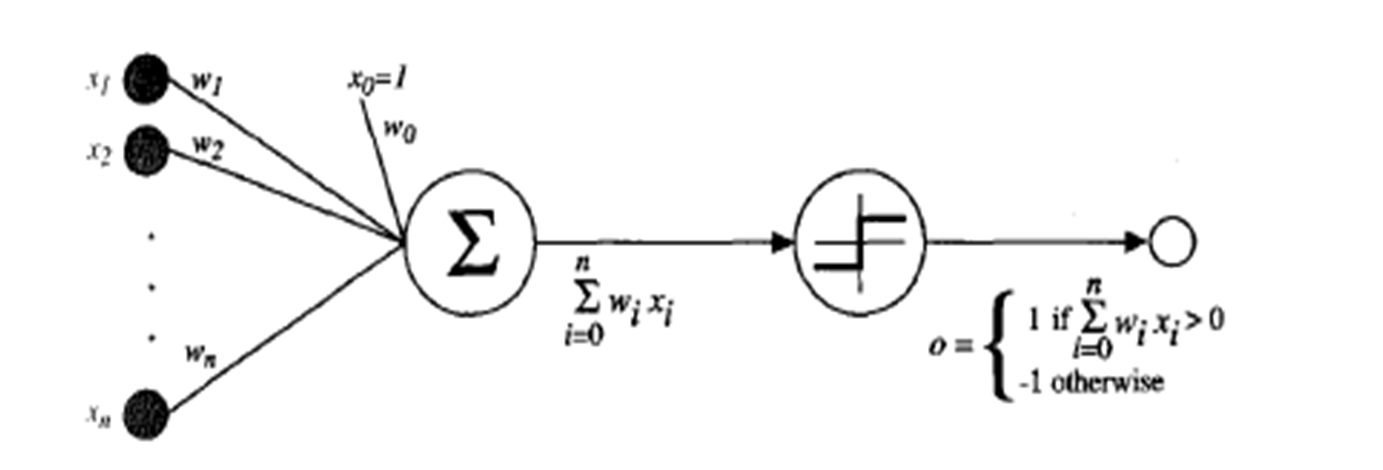### Perceptron

Perceptron

One type of ANN system is based on a unit called a perceptron (also called as linear classifier)

A perceptron takes a vector of real-valued inputs, calculates a linear combination of these inputs, then outputs a 1 if the result is greater than some threshold and -1 otherwisePerceptron Model

More precisely, given inputs xl through x,, the output o(x1, . . . , x,) computed by the perceptron is
o(x1,. ..,xn) = 1 if w0 + wlxl+ w2x2+- - . + WnXn > 0
-1 otherwise

where each wi is a real-valued constant, or weight, that determines the    contribution of input xi to the perceptron output
Notice the quantity (-w0) is a threshold that the weighted combination of inputs wlxl + . . . + wnxn must surpass in order for the perceptron to output a 1